# Mathematics behind K-Mean Clustering algorithm

K-Means is one of the simplest unsupervised clustering algorithm which is used to cluster our data into K number of clusters. The algorithm iteratively assigns the data points to one of the K clusters based on how near the point is to the cluster centroid. The result of K-Means algorithm is:

1. K number of cluster centroids
2. Data points classified into the clusters

#### Applications:

K-Means can be used for any type of grouping where data has not been explicitly labeled. Some of the real world examples are given below:

#### Outline of the algorithm:

Assuming we have input data points x1,x2,x3,…,xn and value of K (the number of clusters needed). We follow the below procedure:

1. Pick K points as the initial centroids from the dataset, either randomly or the first K.
2. Find the Euclidean distance of each point in the dataset with the identified K points (cluster centroids).
3. Assign each data point to the closest centroid using the distance found in the previous step.
4. Find the new centroid by taking the average of the points in each cluster group.
5. Repeat 2 to 4 for a fixed number of iteration or till the centroids don’t change.
###### Euclidean Distance between two points in space:

If p = (p1p2) and q = (q1q2) then the distance is given by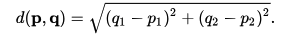Implementation:

def euclidean_distance(point1, point2):
return math.sqrt((point1-point2)**2 + (point1-point2)**2)
###### Assigning each point to the nearest cluster:

If each cluster centroid is denoted by cithen each data point x is assigned to a cluster based on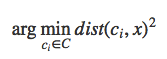here dist() is the euclidean distance

Implementation:

#find the distance between the points and the centroids
for point in data:
distances = []
for index in self.centroids:
distances.append(self.euclidean_distance(point,self.centroids[index]))

#find which cluster the datapoint belongs to by finding the minimum
#ex: if distances are 2.03,1.04,5.6,1.05 then point belongs to cluster 1 (zero index)
cluster_index = distances.index(min(distances))
self.classes[cluster_index].append(point)
###### Finding the new centroid from the clustered group of points: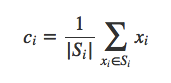Si is the set of all points assigned to the ith cluster.

Implementation:

#find new centroid by taking the centroid of the points in the cluster class
for cluster_index in self.classes:
self.centroids[cluster_index] = np.average(self.classes[cluster_index], axis = 0)

#### K-Means full implementation

The above program creates a sample set for 4 clusters and then performs K-Means on it

#### K-Means implementation using python sklearn:

from sklearn.cluster import KMeans
import numpy as np
import matplotlib.pyplot as plt
from sklearn.datasets.samples_generator import make_blobs

#generate dummy cluster datasets
K = 4
X, y_true = make_blobs(n_samples=300, centers=K,
cluster_std=0.60, random_state=0)
k_means = KMeans(K)
k_means.fit(X)

cluster_centres = k_means.cluster_centers_

y_kmeans = k_means.predict(X)
plt.scatter(X[:, 0], X[:, 1], c=y_kmeans, s=50, cmap='viridis')

for centroid in cluster_centres:
plt.scatter(centroid,  centroid, s=300,  c='black', alpha=0.5)

Output from the above program: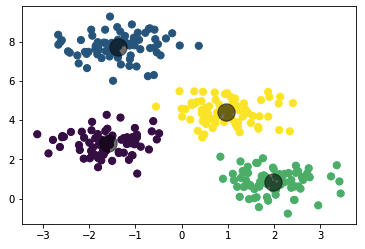The output of the clustering we wrote from scratch is similar to the one we get by using the sklearn library.

#### Choosing Initial centroids

In our implementation we chose the first 4 points as our initial cluster centroids which may give slightly different centroids each time the program is run on random dataset. We can also use the K-means++ method to choose our initial centroids. k-means++ was proposed in 2007 by Arthur and Vassilvitskii. This algorithm comes with a theoretical guarantee to find a solution that is O(log k) competitive to the optimal k-means solution. Sklearn KMeans class uses kmeans++ as the default method for seeding the algorithm.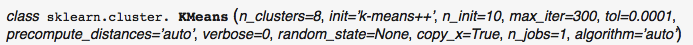0 0 vote
Article Rating
Subscribe
Notify ofInline Feedbacks2 years ago

[…] my previous post to understand how K-Means algorithm works. In this post I will try to run the K-Means on Iris […]Ilya
1 year ago

Thank you much for your explanation, it is very clear!
There is a slight mistake in python code: in the # start K-Mean clustering loops, the nested loop should be looped using another iterator than i. It causes mistakes.[…] distance. Muthu Krishnan does a good job of explaining the math behind the algorithm in this post. To explain the K-means process based on […][…] So far, we have learnt about the introduction to the K-Means algorithm. We have learnt in detail about the mathematics behind the K-means clustering algorithm and have learnt how Euclidean distance method is used in grouping the data items in K number of clusters. Here were are implementing K-means clustering from scratch using python. But the problem is how to choose the number of clusters? In this example, we are assigning the number of clusters ourselves and later we will be discussing various ways of finding the best number of clusters. This code is referenced from Muthu. […]

4
0